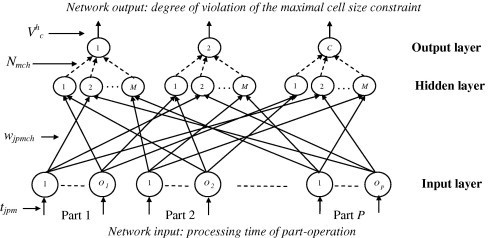# Hopfield network and Boltzmann machine

May 27 • General • 7955 Views • 2 Comments on Hopfield network and Boltzmann machine

HOPFIELD NETWORK:
John J. Hopfield developed a model in the year 1982 conforming to the asynchronous nature of biological neurons. The network proposed by Hopfield are known as Hopfield networks. This network has found many useful application in associative memory and various optimization problems. Two types of network are- discrete and continuous Hopfield networks.

Discrete Hopfield Network:

The Hopfield network is an autoassociative fully interconnected single-layer feedback  network. It is also a symmetrically weighted network. When this operated in discrete line fashion it is called discrete Hopfield network and its architecture as a single-layer feedback network can be called as recurrent. The network takes two valued inputs: binary (0, 1)or bipolar (+1, -1); the use bipolar input makes the analysis easier.

A Hopfield network with binary input vectors is used to determine whether an input vector is a “known” vector or an “unknown” vector. If the input vector is na unknown vector, the activation vector resulted during iteration will converge to an activation vector which is not one of the stored patterns, such a pattern is called as spurious stable state.

Configuration:-Training Algorithm:-

Step 0: initialize the weights to store pattern, i.e., weights obtained from training algorithm using Hebb rule.

Step 1: When the activations of the net are not converged, then perform step 2 to 8.

Step 2: Perform step 3 to 7 for each input vector X.

Step 3: Make the initial activation of the net equal to the external input vector X:’

yi=xi(i=1 to n)

Step 4: Perform step 5 to 7 for each unit Yi.

Step 5: Calculate the net input of the network:

yini=xi + ∑yj.wji

Step 6: Apply the activation over the net input to calculate the output:

Yi = 1, if yini>Өi  or  yi, if yini= Өi  or  0, if yini< Өi

Where Өi is the threshold and is normally taken as zero.

Step 7: Now transmit the obtained output yi to all other units. Thus, the activation vectors are updated.

Step 8: Finally, test the net for convergence.

Continuous Hopfield Network:

A discrete Hopfield net can be modified to a continuous model, in which time is assumed to be a continuous  variable, and can be used for associative memory problems or optimization problems like travelling salesman problem. The continuous Hopfield net can be realized as an electronic circuit, which uses non-linear amplifiers and resistors. This helps building the Hopfield network using analog VLSI technology.

BOLTZMANN MACHINE:
The early optimization technique used in  artificial neural networks is based on the Boltzmann machine.When the simulated annealing process is applied to the discrete Hopfield network, it become a Boltzmann machine. On applying the Boltzmann machine to a constrained optimization problem, the weights represent the constraint of the problem and the quantity to0 be optimized.

The Boltzmann machine consists of  a set of units (Xi and Xj) and a set of bi-directional connections between pairs of units. This machine can be used as an associative memory. The weighs of a Boltzmann machine is fixed; hence there is no specific training algorithm for updation of weights. (For a Boltzmann machine with learning , there exists a training procedure.) With the Boltzmann machine weights remaining fixed, the net  makes its transition toward maximum of the CF.

Algorithm:-

Step 0: Initialize the weights representing the constraint of the problem. Also initialize control parameter T and activate the units.

Step 1: When stopping condition is false, perform step 2 to 8.

Step 2: Perform step 3 to 6 n^2 times.

Step 3: integers I and J are chosen random values between 1 and n.

Step 4: Calculate the change in consensus:

∆CF= (1-2XI,J)[w(I,J:I,J) + ∑∑w(I,j : I, J)XI,J]

Step 5: Calculate the probability of acceptance of the change in state-

AF(T)=1/1 + exp[-(∆CF/T)]

Step 6: Decide whether to accept the change or not. Let R be a random number between 0 and 1. If R<AF, accept the change;

XI,J=1-XI,J

If R≥AF, reject the change.

Step 7: Reduce the control parameter T,

T(new)=0.95 T(old)

Step 8: Test for stopping condition, which is- If the temperature reaches a specified value or if no change of state for specified number of epochs then stop, else continue.

### 2 Responses to Hopfield network and Boltzmann machine

1. Rachita Mishra says:

A Boltzmann machine is a type of stochastic recurrent neural network invented by Geoffrey Hinton and Terry Sejnowski. Boltzmann machines can be seen as the stochastic, generative counterpart of Hopfield nets.Here the detail about this is beautifully explained.

2. Shilpa Ranjan says:

This post explains about the Hopfield network and Boltzmann machine in brief. A step by step algorithm is given for both the topic. This can be a good note for the respective topic.Going through it can be helpful !!!